# An Essential Introduction to Machine Learning## A Step-by-Step Guide to Make Your Computer Learn using Google’s TensorFlow

Machine Learning is all the rage. It’s effectiveness across industries is stunning, and it is rapidly improving. This short guide will allow you to understand the process, time, difficulty, and expected results. Finally, you’ll have the chance of making your machine learn to recognize handwriting.

The goal is to cover a small part of Machine Learning in a sufficiently broad manner to provide to the non-practitioner an insight, a lens through which make decisions.

## What is the Machine Actually Learning?

Machine Learning is very different from how we humans learn. We learn by observing, associating, repeating, abstracting, categorising, reconstructing, making mistakes, using different senses,… We can do it at will by placing our attention to what we want to learn or we can even store something that we have seen just once, per chance, for just a moment. How our brain and body does this exactly remains largely a fascinating mystery.

Machine Learning (as of 2016) also uses a lot of repeating, abstracting, categorising, reconstructing, and making mistakes. However, computers don’t actually observe, they merely sense, because there isn’t yet a very effective system to attend to specific characteristics. Computers also don’t have yet a very effective way of using different senses or associating different elements. Machines need a lot of examples, a lot of time to train (1–2 weeks, depending on the task), and once the training is done, they don’t learn any more, at all.

## The Underlying Principle of How Machine Learning Works

Machine Learning tries to find one single mathematical formula that takes the input (e.g., an image, a sound), and transforms it into a probability that it belongs to a trained category. The underlying principle is that a sufficiently complex formula can capture the common characteristics while filtering out the differences.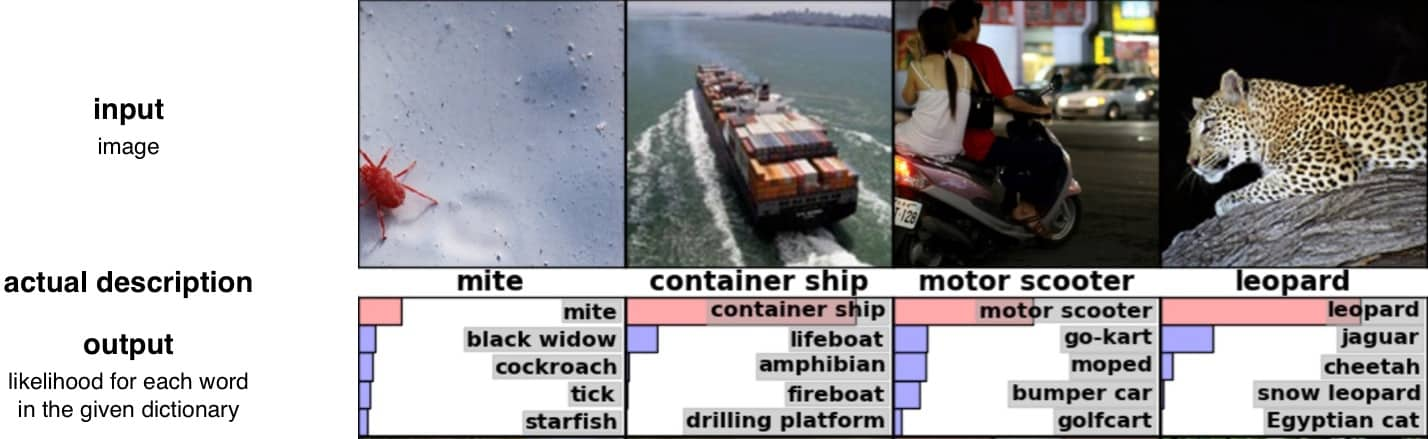The most effective structure that scientists have found this far is representing the formula as a network of connected elements, a so-called neural network. Each element — an artificial, simplified neuron — processes the input data from one or more sources by using simple operations such as addition and multiplication. The machine learns how these artificial neurons are connected and how they process the information.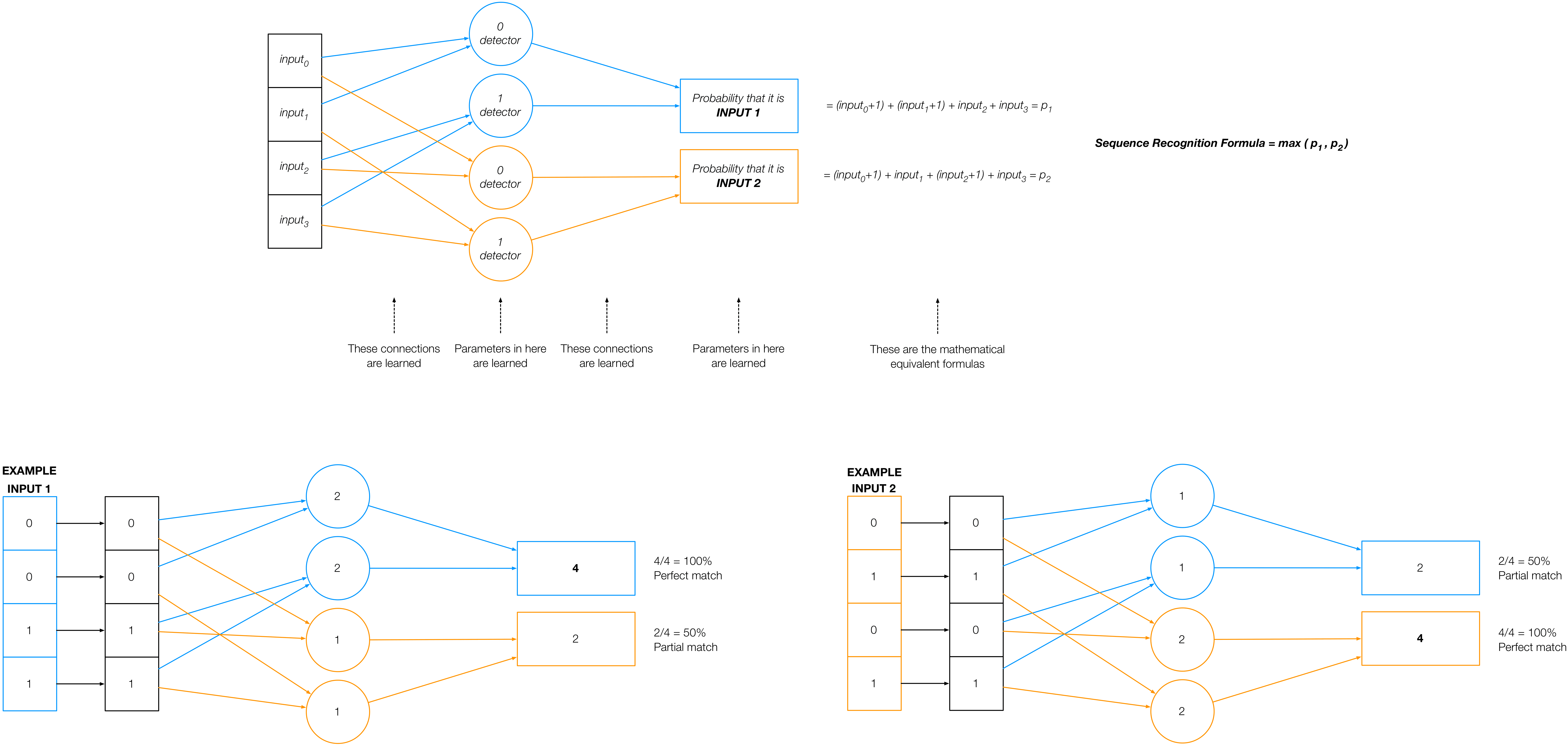A typical network contains many many neurons — millions. The large amount of parameters is why it takes so long to train a neural network. The actual size of the network depends on the application, and although the theory is not yet conclusive, it has been found that a large network is more robust in recognize targets over a wide variety of inputs. This also shows that Machine Learning — despite the name — depends a lot on humans giving it a structure (e.g., the number of neurons in the example above), and appropriate training samples to learn.

## The Machine Learning Process

1. Architecture
The human defines how the network looks and the rules for learning.
2. Training
The machine analyses training data and adjusts the parameters, trying to find the best possible solution in the given architecture.
3. Usage
The network is “frozen” (i.e., it doesn’t learn any more), and is fed with actual data to obtain the desired outcome.

## Process Step 1: ARCHITECTURE

The human defines:
– Number of neurons
– Number of layers
– Sampling sizes
– Convolution sizes
– Pooling sizes
– Neural response function (e.g., – ReLU)
– Error function (e.g., cross-entropy)
– Error propagation mechanism
– Number of iterations
– And many more parameters…

How are these characteristics decided? Part theory, part experience, part trial and error.  The result of any Machine Learning system is only as good as the choice of its architecture.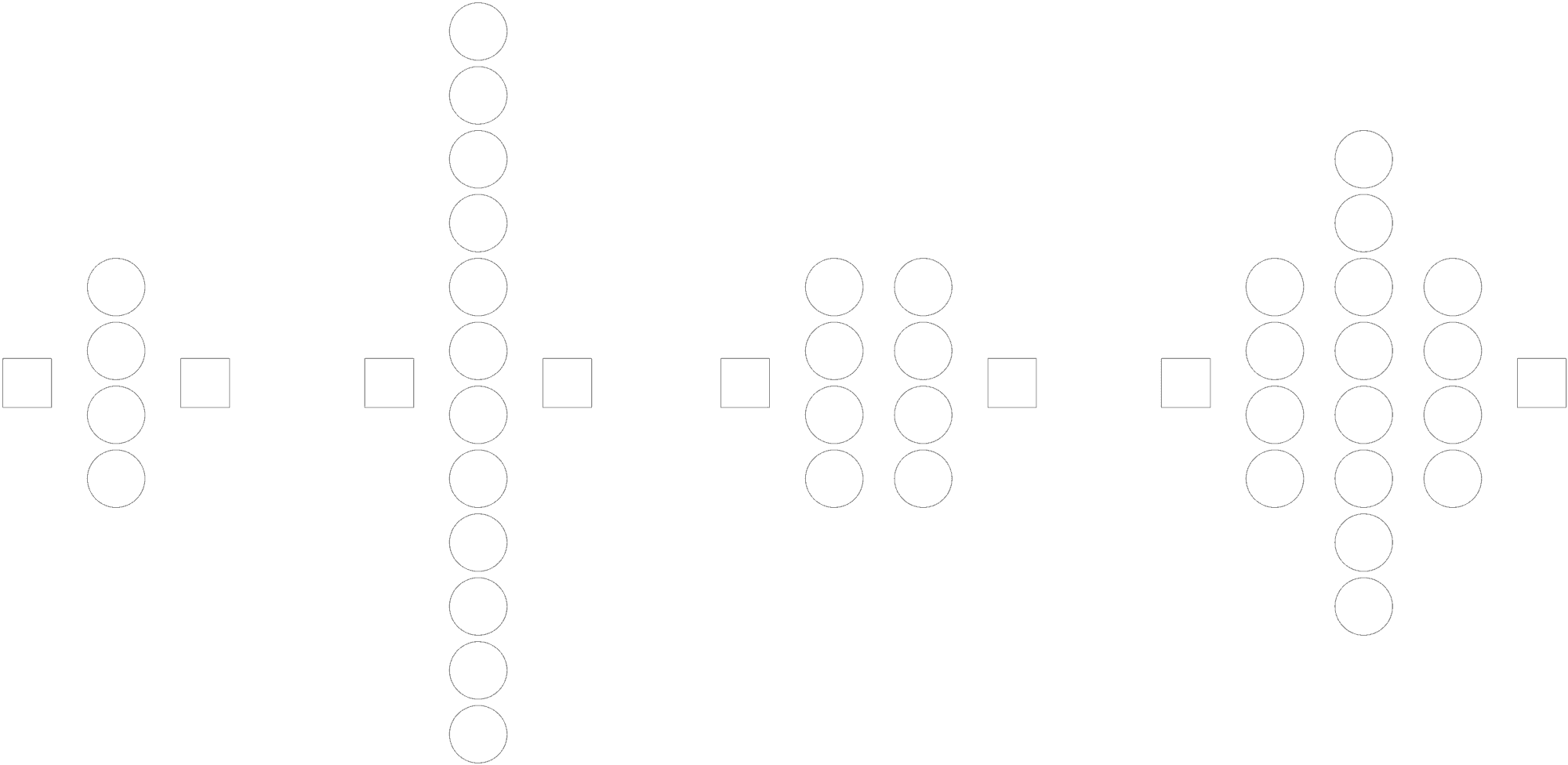What is crucial about the architecture, is that it must allow for efficient training. For instance, the network must allow for an error signal to affect its individual components. Part of the reason why Machine Learning systems are becoming widely successful is that scientists such as Hinton, LeCun, and Bengio have found ways of training complex networks.

## Process Step 2: TRAINING

The neural network starts off with semi-random parameters, and then the computer iteratively improves them to minimize the difference — the error — between the inputs and the output. A typical procedure is the following:

1. Calculate the output of the neural network from a training input
2. Calculate the difference between the expected and the actual output
3. Change input, and carry out steps (1) and (2) again
4. Adjust the parameters of the neurons (e.g., slightly decrease the weight of a neuron if the output difference has increased) and of the network (e.g., freeze certain layers)
5. Start again from (1)

After a number of iterations (chosen as part of the architecture), the overall performance is calculated, and if sufficient, the artificial neural network is ready to be deployed.

What if the system doesn’t produce the desired outcome? Back to square 1: Architecture. There is not yet a method to tweak the parameters consequently to improve the system. What this highlights once again is that Machine Learning is a Swiss Army knife, but it’s up to the user to decide which tool to use, how, and when.

## Process Step 3: USAGE

Using a Machine Learning system consists in providing it with an input, and gathering the result. There is no more learning and very few parameters can be changed, if any at all.
The processing speed depends on the complexity of the network, the efficiency of the code, and the hardware. Machine Learning has profited immensely from the gaming industry, which has spearheaded the development of increasingly powerful GPUs. Indeed, the Graphical Processing Units — originally used to display images on a screen — could be modified to carry out both the training and the production usage of neural networks. The underlying reason is that GPUs are capable of executing many simple operations in parallel, such as calculating the result of the interaction between individual neurons. Calculating millions of interactions at the same time instead of one after the other is a key advantage over other systems.

## Now Try It Yourself A step-by-step guide to running Google TensorFlow Machine Learning on your computer

Here is a simple example that you can try to run to get a feeling of what it means to architect, train, and use a Deep Learning network, right on your computer (as of 2016).
The application we will train is recognizing hand-written digits. This was one of the original problems of AI research, and it had concrete industrial applications in the early days of digitalisation (read more about digital strategy here). For instance for recognizing amounts on banking cheques or addresses on mail envelopes.

The Machine Learning framework we will use is Google’s Tensorflow (tested on r0.7).
The steps are intended for a Mac (tested on OSX El Capitan 10.11.4 with Python 2.7). Instructions for Linux/Ubuntu are available here.

## Installation

Launch terminal from spotlight (press ⌘–space on the keyboard and then write terminal, press enter). When the terminal window has opened, copy and paste the following commands (you’ll be asked your password):

```sudo easy_install pip

virtualenv --system-site-packages ~/tensorflow

source ~/tensorflow/bin/activate

pip install jupyter

cd tensorflow

jupyter notebook```

This will open a tab in the browser (if not, troubleshoot here), from which you can create an interactive “notebook” by clicking on the spot indicated by the red arrow: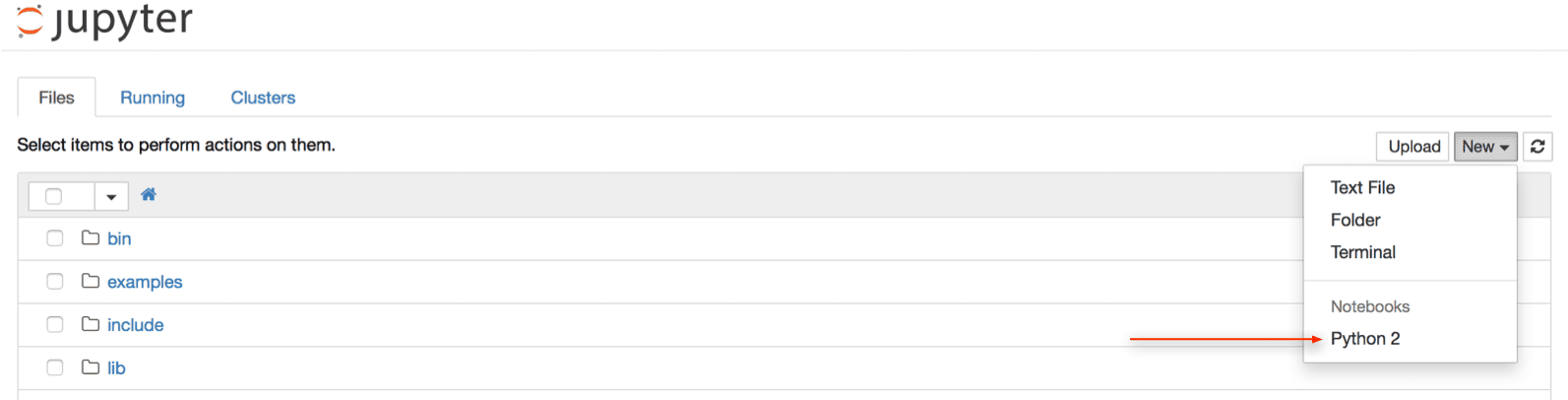The Python interactive notebook will open and looks like this: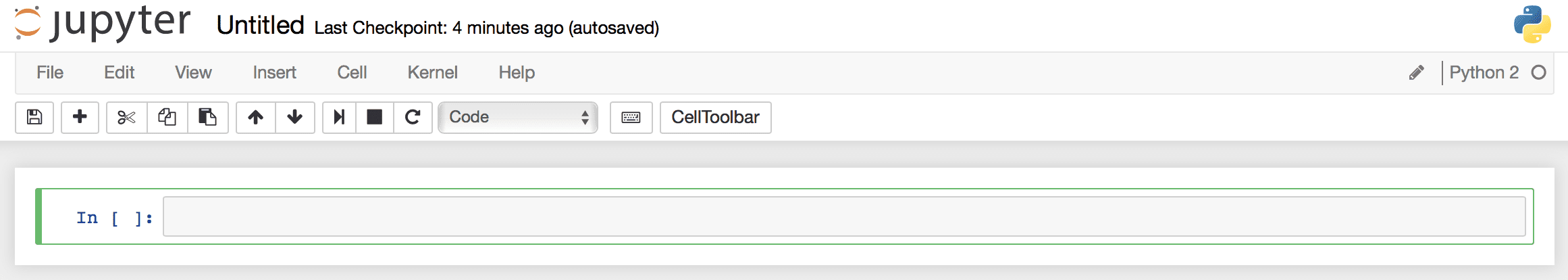Write in the box, then click on ⇥ to run (or hit shift-enter) to see the results.

## Setup of the Machine Learning environment for recognising digits

First, add TensorFlow and other necessary components by copy-and-paste of the following in your Python notebook:

```import numpy as np
import matplotlib as mp
%matplotlib inline
import matplotlib.pyplot as plt
import tensorflow as tf
sess = tf.Session()

def getActivations(layer,stimuli):
units = layer.eval(session=sess,feed_dict={x:np.reshape(stimuli,[1,784],order='F'),keep_prob:1.0})
plotNNFilter(units)

def plotNNFilter(units):
filters = units.shape
plt.figure(1, figsize=(20,20))
for i in xrange(0,filters):
plt.subplot(7,6,i+1)
plt.title('Filter ' + str(i))
plt.imshow(units[0,:,:,i], interpolation="nearest", cmap="gray")```

Then import the training and test data

```from tensorflow.examples.tutorials.mnist import input_data

We can take a look what the images look like:

```imageWidth = imageHeight = 28

testImageNumber = 1 # Change here to see another

imageToUse = mnist.test.images[testImageNumber]
plt.imshow(np.reshape(imageToUse,[imageWidth,imageHeight]), interpolation="nearest", cmap="gray_r")```

## Machine Learning Process Step 1: ARCHITECTURE

Now is the time to start setting the basis of the machine learning by defining fundamental computations.
What is interesting to note is that the 2D structure of the images is flattened into a 1D vector, because in this learning framework it doesn’t matter.

```inputVectorSize = imageWidth*imageHeight

numberOfPossibleDigits = 10 # handwritten digits between 0 and 9
outputVectorSize = numberOfPossibleDigits

x = tf.placeholder(tf.float32, [None, inputVectorSize],name="x-in")
y_ = tf.placeholder(tf.float32, [None, outputVectorSize],name="y-in")

def weight_variable(shape):
initial = tf.truncated_normal(shape, stddev=0.1)
return tf.Variable(initial)

def bias_variable(shape):
initial = tf.constant(0.1, shape=shape)
return tf.Variable(initial)

def conv2d(x, W):
return tf.nn.conv2d(x, W, strides=[1, 1, 1, 1], padding='SAME')
# overlapping strides (2: non-overlapping)

def max_pool_2x2(x):
return tf.nn.max_pool(x, ksize=[1, 2, 2, 1],strides=[1, 2, 2, 1], padding='SAME')```

Next, we set the layers to be trained, and how to calculate the final probability. As you can see, it’s a convolutional neural network (a.k.a., ConvNet) with a rectifying neuron (ReLU). In this case, the output is calculated using a normalised exponential, the softmax function.

```outputFeatures1 = 4
outputFeatures2 = 4
outputFeatures3 = 16

# Input
x_image = tf.reshape(x, [-1,imageWidth,imageHeight,1])

# Individual neuron calculation: y = conv(x,weight) + bias

# Layer 1: convolution
W_conv1 = weight_variable([5, 5, 1, outputFeatures1])
b_conv1 = bias_variable([outputFeatures1])
h_conv1 = tf.nn.relu(conv2d(x_image, W_conv1) + b_conv1)
h_pool1 = max_pool_2x2(h_conv1)

# Layer 2: convolution
W_conv2 = weight_variable([5, 5, outputFeatures1, outputFeatures2])
b_conv2 = bias_variable([outputFeatures2])
h_conv2 = tf.nn.relu(conv2d(h_pool1, W_conv2) + b_conv2)
h_pool2 = max_pool_2x2(h_conv2)

# Layer 3: convolution
W_conv3 = weight_variable([5, 5, outputFeatures2, outputFeatures3])
b_conv3 = bias_variable([outputFeatures3])
h_conv3 = tf.nn.relu(conv2d(h_pool2, W_conv3) + b_conv3)

# Layer 4: Densely connected layer
W_fc1 = weight_variable([7 * 7 * outputFeatures3, 10])
b_fc1 = bias_variable()
h_conv3_flat = tf.reshape(h_conv3, [-1, 7*7*outputFeatures3])
keep_prob = tf.placeholder("float")
h_conv3_drop = tf.nn.dropout(h_conv3_flat, keep_prob)

# Output
y_conv = tf.nn.softmax(tf.matmul(h_conv3_drop, W_fc1) + b_fc1)```

Then we define the method to adjust the parameters and what kind of difference between expected and actual output we want to use (in this case, cross-entropy).

```cross_entropy = -tf.reduce_sum(y_*tf.log(y_conv))
correct_prediction = tf.equal(tf.argmax(y_conv,1), tf.argmax(y_,1))
accuracy = tf.reduce_mean(tf.cast(correct_prediction, "float"))

What TensorFlow actually does here, behind the scenes, is it adds new operations to your graph which implement backpropagation and gradient descent.

## Machine Learning Process Step 2: TRAINING

We’re now ready to let the computer learn to classify the image inputs into numbers from 0 to 9.

```sess.run(tf.initialize_all_variables())

iterations = 0
trainingImageBatchSize = 50

for iterations in range(0,1000):
batch = mnist.train.next_batch(trainingImageBatchSize)
train_step.run(session=sess, feed_dict={x:batch,y_:batch, keep_prob:0.5})

if iterations%100 == 0:
trainAccuracy = accuracy.eval(session=sess, feed_dict={x:batch,y_:batch, keep_prob:1.0})
print("step %d, training accuracy %g"%(iterations, trainAccuracy))```

You’ll see that it takes quite some time to train (a few minutes), despite the small images and network. The more iterations, the better the accuracy, possibly (because it partially depends on the semi-random initialisation values) reaching a peak before 1000 iterations. While you wait for the results, ponder about the fact that you don’t see any of the values of the neurons, and that ultimately this doesn’t matter.

When the machine is done learning, we can take a look at the different layers to see what they are calculating:

```testImageNumber = 1 # Change here to use another

imageToUse = mnist.test.images[testImageNumber]

getActivations(h_conv1,imageToUse)

You can also try these:

getActivations(h_conv2,imageToUse)

getActivations(h_conv3,imageToUse)```

## Machine Learning Process Step 3: USAGE

Finally, let’s see how well the network your computer learned is able to recognize all the handwritten digits in the dataset.

```testAccuracy = accuracy.eval(session=sess, feed_dict={x:mnist.test.images,y_:mnist.test.labels, keep_prob:1.0})

print("test accuracy %g"%(testAccuracy))```

Congratulations! You taught your computer to recognize handwritten digits.
If you wish, you can go further and customise the system to use your own handwriting.

## Cleanup and Finish

When you’re done, go back to the Terminal, hit twice Ctrl-C to exit Jupiter, then type:

`deactivate`

Then ⌘–q to quit the terminal.

Start Again

To try out something else next time, the procedure is easier. Just copy and paste the following:

```source ~/tensorflow/bin/activate

cd tensorflow

jupyter notebook```